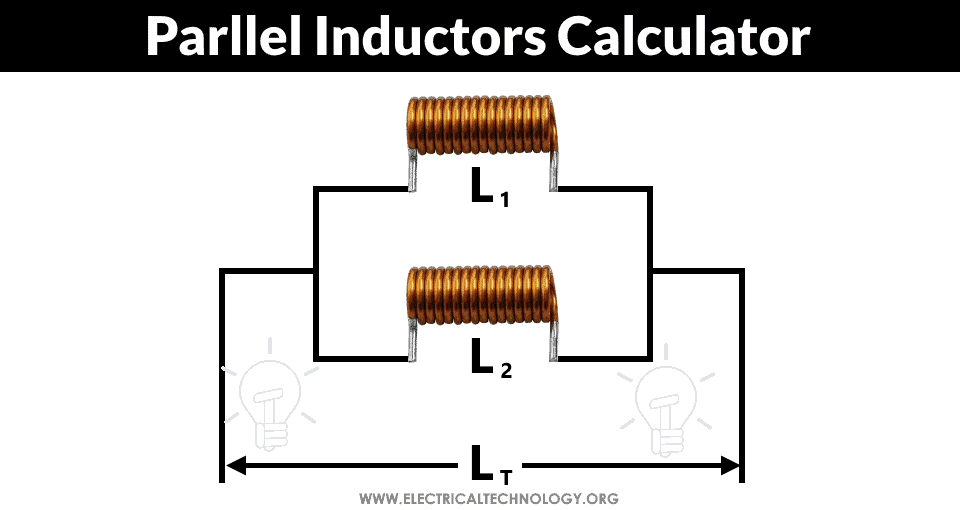# Parallel Inductors Calculator

## Inductance of Parallel Connected Inductors Calculator

### Formula & Equations for Parallel Inductors Calculator

Finding Total Inductance (LT) when L1 and L2 are given;

1/LT = 1/L1 + 1/L2

LT = L1||L2

LT = L1 x L/ (L1 + L2)

Finding L1 when LT and L2 are given;

L1 = L2 × LT / (L2 – LT)

Finding L2 when LT and L1 are given;

L2 = L× R/ (L1 – LT)### Parallel Inductors Calculator

Enter any two values (inductance of inductors) and Select Unit then Click on Calculate. Result will display the required Value.

 Enter Values Inductor L1: µHmHHenryKHMH Inductor L2: µHmHHenryKHMH Parallel Inductance LT: µHmHHenryKHMH

Related Calculators: